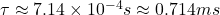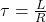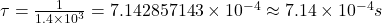A resistor, inductor, and a battery are arranged in a circuit. The circuit has an inductance of L = 1 H and a resistance of 1.4 kΩ. Switch S

Question

A resistor, inductor, and a battery are arranged in a circuit. The circuit has an inductance of L = 1 H and a resistance of 1.4 kΩ. Switch S1 is suddenly closed at t = 0. Find the time needed for the current to reach a fraction f = 0.6 of its maximum value.

in progress 0
6 months 2021-08-04T07:22:39+00:00 1 Answers 17 views 0